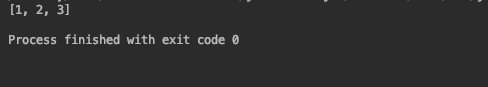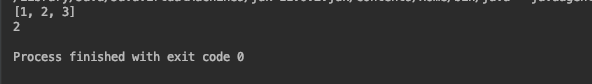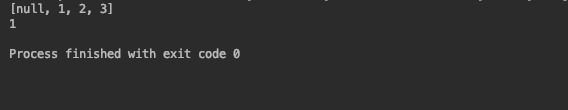tag:www.digitalocean.com,2005:/community/tags/set.atom DigitalOcean Community | Set tutorials, questions and resources 2023-05-31T16:07:08.255Z DigitalOcean <![CDATA[How to Convert Set to List in Java]]> tag:www.digitalocean.com,2005:/community/tutorials/set-to-list-in-java 2022-08-03T17:52:17.919Z Lists in Java are ordered collection of data, whereas sets are an unordered collection of data. A list can have duplicate entries, a set can not. Both the data structures are useful in different scenarios.

Knowing how to convert a set into a list is useful. It can convert unordered data into ordered data.

## Initializing a set

Let’s initialize a set and add some elements to it.

``````import java.util.*;

public class Main {

public static void main(String[] args)
{
Set<Integer> a = new HashSet<>();
System.out.println(a);
}
}
``````The add() method of HashSet adds the elements to the set. Note that the elements are distinct. There is no way to get the elements according to their insertion order as the sets are unordered.

## Converting Set to List in Java

Let’s convert the set into a list. There are multiple ways of doing so. Each way is different from the other and these differences are subtle.

### 1. List Constructor with Set argument

The most straightforward way to convert a set to a list is by passing the set as an argument while creating the list. This calls the constructor and from there onwards the constructor takes care of the rest.

``````import java.util.*;

public class Main {

public static void main(String[] args)
{
Set<Integer> a = new HashSet<>();

List<Integer> arr = new ArrayList<>(a);
System.out.println(arr);
System.out.println(arr.get(1));

}
}
``````

Since the set has been converted to a list, the elements are now ordered. That means we can use the get() method to access elements by index.

### 2. Using conventional for loop

We can use the good old for loop to explicitly copy the elements from the set to the list.

``````import java.util.*;

public class Main {

public static void main(String[] args)
{
Set<Integer> a = new HashSet<>();

List<Integer> arr = new ArrayList<>(a);
for (int i : a)
System.out.println(arr);
System.out.println(arr.get(1));
}
}
``````For loop goes over the set element by element and adds the elements to the list.

Lists have a method called addAll() that adds multiple values to the list at once. You might recall this operation from its use in merging two lists. addAll() also works for adding the elements of a set to a list.

``````import java.util.*;

public class Main {

public static void main(String[] args)
{
Set<Integer> a = new HashSet<>();

List<Integer> arr = new ArrayList<>();
System.out.println(arr);
System.out.println(arr.get(1));
}
}
``````### 4. Stream API collect() method

Stream.collect() is available from Java 8 onwards. ToList collector collects all Stream elements into a List instance.

``````import java.util.*;
import java.util.stream.Collectors;

public class Main {

public static void main(String[] args)
{
Set<Integer> a = new HashSet<>();

List<Integer> arr;

arr = a.stream().collect(Collectors.toList());
System.out.println(arr);
System.out.println(arr.get(1));
}
}
``````The documentation for stream.collect() mentions that there is no guarantee on the type, mutability, serializability, or thread-safety of the List returned. If more control over the returned List is required, use toCollection(Supplier).

To specify the type of list use toCollection(ArrayList::new)

``````import java.util.*;
import java.util.stream.Collectors;

public class Main {

public static void main(String[] args)
{
Set<Integer> a = new HashSet<>();

List<Integer> arr;

arr = a.stream().collect(Collectors.toCollection(ArrayList::new));
System.out.println(arr);
System.out.println(arr.get(1));
}
}
``````

### 5. List.copyOf() method

Java 10 onwards List has a copyOf() method. The method returns an unmodifiable List containing the elements of the given Collection, in its iteration order. The list can’t contain any null elements. In case the set contains ‘null’, the method returns a null pointer exception.

``````import java.util.*;
import java.util.stream.Collectors;

public class Main {

public static void main(String[] args)
{
Set<Integer> a = new HashSet<>();

List<Integer> arr;

arr = List.copyOf(a);
System.out.println(arr);
System.out.println(arr.get(1));
}
}
``````Adding a null in the set and trying to convert to a list :

``````import java.util.*;
import java.util.stream.Collectors;

public class Main {

public static void main(String[] args)
{
Set<Integer> a = new HashSet<>();

List<Integer> arr;

arr = List.copyOf(a);
System.out.println(arr);
System.out.println(arr.get(1));
}
}
``````

If you try to add an element to an immutable list you’ll get an error that looks like the following.

Using the addAll() method to convert set with null element to a list :

``````import java.util.*;
import java.util.stream.Collectors;

public class Main {

public static void main(String[] args)
{
Set<Integer> a = new HashSet<>();

List<Integer> arr = new ArrayList<>();

//  arr = List.copyOf(a);
System.out.println(arr);
System.out.println(arr.get(1));
}
}
``````Note that the null appears at the beginning of the list.

## Conclusion

We saw some really interesting methods to convert a set into a list. It’s important to pay attention to the type of list that is created from each method. Like copyOf() method produces an immutable list and can’t handle null elements. Whereas, stream.collect() doesn’t guarantee anything. Constructor and addAll() are the most trustworthy among the batch.

]]>
Jayant Verma 2022-08-03T17:52:17.919Z